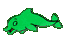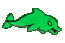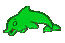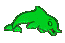Probability of a Single Event     Math Help     Game Tips:

- A 50 percent chance of rain tomorrow means the probability of rain tomorrow is 0.5 or 1/2.
Probability can be represented by a percent, decimal or fraction.

- Zero Probability = 0% = 0.0 = 0/1 means 'no chance' = 'impossible'.
One hundred percent Probability = 100% = 1.0 = 1/1 means 'it will happen for sure'.

- If your name is entered 20 times in a random contest with 80 other names, then the chance
of your name being the one name chosen from the 100 equally likely names is 20/100 = 0.2 = 20%.

- In general for equally likely events, a probability P equals the number of favourable outcomes
divided by the total number of possible outcomes.

- Your Game Score is reduced by the number of dolphin hits.

- To slow the game speed repeat tap/click on the word Slider.
- To increase the game speed repeat tap/click on the word Math.
- Speed can also be adjusted with a keyboard's - and + keys.

- Refresh/Reload the web page to restart the game.